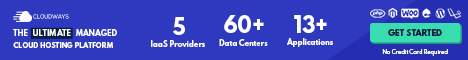# Economic Models II

## Forming and Testing Hypothesis in Economic Models

A Hypothesis in an economic model is a statement that may be either correct or incorrect about an economic variable.

Economic variable is something measurable that relates to resource use that can have different values, for example wages, prices, liters of water.

An economic variable is something measurable that can have different values, such as the price paid for water use.

An example of a hypothesis in an economic model is the statement that ‘charging more for water will lead to a decline in water usage.’

An economic hypothesis is usually about a causal relationship; in this case, the hypothesis states that a higher water price causes, or leads to, reduced amounts of water usage.

Before accepting a hypothesis we must test it. To test a hypothesis we must analyze statistics on the relevant economic variables.

In this case, we must gather statistics on the volume of water used over time and examine how usage changed when the price of water changed. Data may be required on other variables as well.Testing a hypothesis can be tricky.

For example, showing that higher prices for water have in the past led to lower amounts being used would not be enough to demonstrate that the higher prices caused a reduction in water usage.

Just because two things are correlated—that is, they are associated with each other—does not mean that one caused the other.

For example, suppose that in the past the government had increased the price of water but at the same time rainfall increased, reducing the need for as much irrigation from rivers and dams. Or suppose that biological engineering had developed varieties of crops that didn’t require as much water.

Over a period of time, many economic variables will be changing, which complicates testing hypotheses.

In fact, when economists disagree about a hypothesis it is often because of disagreements over interpreting the statistical analysis used to test the hypothesis.

Note that hypotheses must be statements that could in principle turn out to be incorrect. Statements such as ‘reduction in taxation rates are good’ or ‘reductions in taxation rates are bad’ are value judgments, rather than hypotheses, because it is not possible to prove or disprove them.

Economists accept and use an economic model if it leads to hypotheses that can be confirmed by statistical analysis.

In many cases the acceptance is tentative, however, pending the gathering of new data or further statistical analysis.

In fact, economists often refer to a hypothesis having been ‘not rejected’, rather than being ‘accepted,’ by statistical analysis. But what if statistical analysis clearly rejects a hypothesis?

For example, what if the model leads to a hypothesis that changing the price of water does not reduce usage?

It may be that an assumption used in the model was too simple or too limiting.

For example, perhaps the model we used to determine the effect of pricing on water usage did not distinguish between water used for domestic purposes and water used by industry.

If, in fact, we distinguished between alternative uses, we might have found that water used for domestic purposes was affected less by price changes than water used for industrial purposes.

This difference may explain why our hypothesis was rejected by the economic statistics.

The process of developing models, testing hypotheses and revising models occurs not just in economics but also in disciplines such as physics, chemistry and biology. It is often referred to as the scientific method.

Economics Opens in new window is a social science because it applies the scientific method to the study of the interactions between individuals.